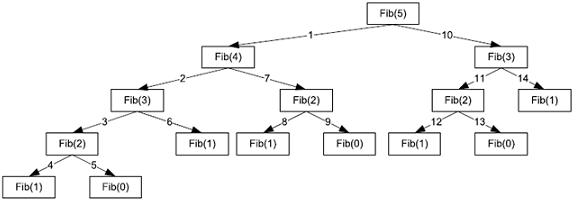# Visual Basic 2010 (Console) GuideRecursive Procedures & Functions

A procedure is recursive if the procedure contains a call to itself. This is perfectly legitimate in Visual Basic and most other high-level languages. A characteristic of recursive procedures is that there must be a stopping condition that can always be reached by the procedure regardless of the input.

The following procedure will calculate the factorial of a number (3! = 3 x 2 x 1 = 6) using a recursive technique.

```Function RecursiveFactorial(ByVal f As Integer) As Integer    If f = 1 Then       Return 1    Else       Return f * RecursiveFactorial(f - 1)    End If End Function Sub Main()    Console.WriteLine(RecursiveFactorial(5))    Console.ReadLine() End Sub```

The function works quite logically. The factorial of a number is the number multiplied by the factorial of the number minus 1. ( 3! = 3 x 2! )

The alternative to recursion is iteration. The factorial function can be re-written using an iterative approach.

```Function IterativeFactorial(ByVal f As Integer) As Integer    Dim total As Integer = 1    For count = 1 To f       total *= count    Next    Return total End Function```

In this example, there is little to choose between the two approaches. The recursive function works a little more like our brain does and is concise. The iterative function is a teeny bit longer but no harder to understand.

## Fibonacci Numbers - The Revenge Of Recursion

Fibonacci numbers are numbers in the following sequence,

0, 1, 1, 2, 3, 5, 8, ...

After 0 and 1, each number is the sum of the previous two numbers in the series. The following pseudocode functions illustrate the difference between a recursive and iterative solution.

### Fibonacci - Recursive

```function fib1(n)    if n = 0: return 0    if n = 1: return 1    return fib1(n - 1) + fib1(n - 2)End Function```

### Fibonacci - Iterative

```function fib2(n)    if n = 0: return 0    if n = 1: return 1    create an array f[0 � n]    f = 0, f = 1    for i = 2 � n:    f[i] = f[i -1] + f[i - 2]    return f[n]End Function```

On the face of it, you'd think that the recursive function would be better. There are fewer lines of code and they are very similar to the steps that we would follow as humans doing the same calculation. You'd be wrong though - there is a major difference between the two approaches.

In the recursive function, every time that a recursive call is made, the program branches to the new function call. Since the program was in the middle of executing another call to the same function, it needs to store the necessary information to allow it to return to the point where it branched. This information is stored in a data structure called a stack and is placed on the top of the stack. When the program has finished executing the new function, it returns to the stack, finds the values it was working with before and completes the unfinished function. In this function, there are lots of branches to place in the stack before returning and this slows the program down more than you might think.

The following program code contains VB implementations of the two functions described above. It uses the StopWatch class to time the execution of each algorithm.

```Sub Main()    Dim numToFind As Integer    Console.Write("Enter the term you want to find ")    numToFind = Console.ReadLine()    Dim stopW As Stopwatch = New Stopwatch()    Dim term As Long    stopW.Reset()    stopW.Start()    term = IterativeFibonacci(numToFind)    stopW.Stop()    Console.WriteLine("Term no {0} = {1} Iterative: {2} Milliseconds", numToFind, term, stopW.ElapsedMilliseconds)    stopW.Reset()    stopW.Start()    term = RecursiveFibonacci(numToFind)    stopW.Stop()    Console.WriteLine("Term no {0} = {1} Recursive: {2} Milliseconds", numToFind, term, stopW.ElapsedMilliseconds)    Console.ReadLine() End Sub Function RecursiveFibonacci(ByVal a As Integer) As Long    If a = 0 Then Return 0    If a = 1 Then Return 1    Return RecursiveFibonacci(a - 1) + RecursiveFibonacci(a - 2) End Function Function IterativeFibonacci(ByVal a As Integer) As Long    If a = 0 Then Return 0    If a = 1 Then Return 1    Dim fibNums(a + 1) As Long    fibNums(0) = 0    fibNums(1) = 1    For count = 2 To a       fibNums(count) = fibNums(count - 1) + fibNums(count - 2)    Next    Return fibNums(a) End Function```

Copy and compile this program. Enter small numbers like 10 or 20 and you won't see a great deal of difference between the two functions. Crank things up to 50 or so and then you'll start to notice that there is a large difference between these two approaches. The problem is the number of function calls that are required by the recursive program. The following tree diagram shows the function calls for the 5th term of the sequence.As n increases, the number of function calls quickly gets out of hand. If you look at the following table, you can see that by around n=10, the number of function calls is already very high. By n=20, the number of function calls is over 20000.

n Recursive Calls
2 2
3 4
4 8
5 14
6 24
7 40
8 66
9 108
10 176

Each function call requires at least one line of code to be executed, maybe more. Each time n increases, the recursive procedure requires lots more lines of code. The iterative procedure increases by 1 loop iteration as n increases.